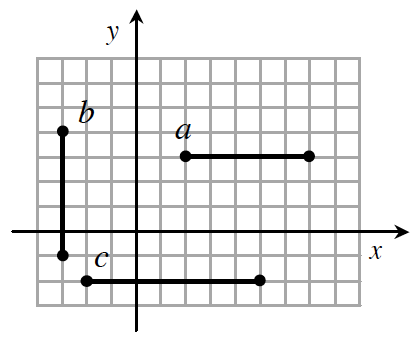### Home > GC > Chapter 7 > Lesson 7.3.1 > Problem7-102

7-102.The diagram at right shows three bold segments. Find the coordinates of the midpoint of each segment.

Start at the endpoints of each segment and move to the center for each segment. What are the coordinates of this point? In order to calculate the coordinates of the midpoint, average the $x$-coordinates and the $y$-coordinates of the endpoints.